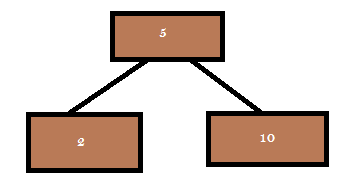# Double the binary tree with the example in C++

Double the binary tree with the example in Cpp:

For each node in a binary search tree, create a new duplicate node, and insert the duplicate as the left child of the original node.
The resulting tree should still be a binary search tree.

See the below sample treeSample tree

After doubling the tree the result isDouble the tree

As we saw the mirror of the tree wich is implemented without changing the root node pointer, similarly this can be accomplished.

The Function prototype is

` void doubleTree(struct pstNode* pstTempNode)`

```void doubleTree(struct pstNode* pstTempNode)

{
struct pstNode* oldLeftNode;
if (pstTempNode==NULL)
return;
// do the subtrees
doubleTree (pstTempNode->left);
doubleTree (pstTempNode->right);
// duplicate this pstTempNode to its left
oldLeftNode = pstTempNode->left;
pstTempNode->left = CreateNode (pstTempNode->data);
pstTempNode->left->left = oldLeftNode;
}

```